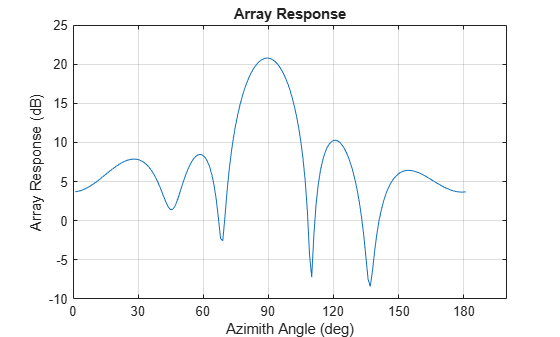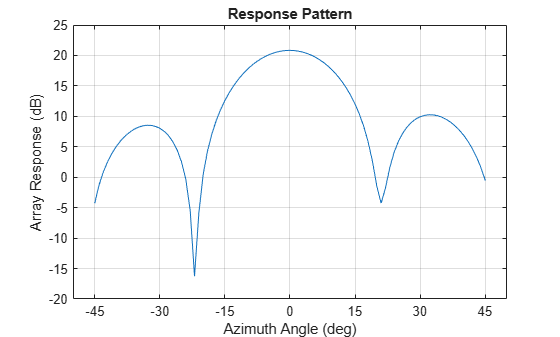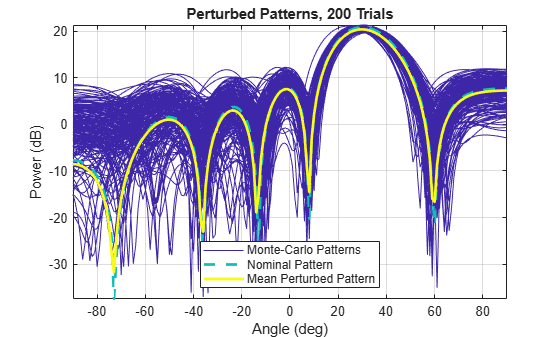# perturbedPattern

Display pattern of perturbed array

Since R2022a

## Syntax

``pmc = perturbedPattern(array,freq)``
``pmc = perturbedPattern(array,freq,az)``
``pmc = perturbedPattern(array,freq,az,el)``
``pmc = perturbedPattern(___,Name=Value)``
``[pmc,pnm,mpmc,varpmc] = perturbedPattern(array,___)``
``perturbedPattern(array,___)``

## Description

````pmc = perturbedPattern(array,freq)` returns a set of perturbed azimuth array patterns `pmc` for an `array` generated from 100 Monte-Carlo runs. `freq` specifies the operating frequency at which the pattern is computed.```

example

````pmc = perturbedPattern(array,freq,az)` also specifies the azimuth angles `az` used for computing the perturbed patterns.```
````pmc = perturbedPattern(array,freq,az,el)` also specifies the elevation angles `el` used for computing the perturbed patterns.```

example

````pmc = perturbedPattern(___,Name=Value)` also sets the specified parameter Name to the specified Value. You can specify additional name-value pair arguments in any order as (Name1=Value1,...,NameN=ValueN). For example, `NumTrials = 10000`.```
````[pmc,pnm,mpmc,varpmc] = perturbedPattern(array,___)` also returns the nominal array response pattern `pnm`, the mean array response pattern `mpmc`, and the variance of the array response pattern `varpmc`.```
````perturbedPattern(array,___)` plots all perturbed patterns for all Monte-Carlo runs and overlays them with both the nominal array response pattern and the mean perturbed array response pattern.```

## Examples

collapse all

Create an 11-element Uniform Linear Array. The array operates at 300 MHz and its elements are spaced one-half wavelength apart. Perturb the element positions by 1/20th of a wavelength. Use the default value of 100 Monte Carlo runs. Compute the pattern for the first simulation at all azimuth angles. Set the random number generator seed for reproducibility.

```freq = 300.0e6; lambda = physconst('LightSpeed')/freq; d = lambda/2; array = phased.ULA(11,ElementSpacing=d/2); rng(10007) perturbations(array,'ElementPosition','Normal',0,lambda/20); perturbations(array,'TaperMagnitude','Normal',0,0.1); pmc = perturbedPattern(array,freq);```

Plot the array pattern vs azimuth angle.

```plot(mag2db(abs(pmc(:,1)))) ylabel('Array Response (dB)') xlabel('Azimith Angle (deg)') title('Array Response') grid on xticks([0:30:180])```Create an 11-element uniform linear array (ULA). The array operates at 300 MHz and its elements are spaced one-half wavelength apart. Perturb the element positions by 1/20th of a wavelength. Use the default value of 100 Monte Carlo runs. Compute the pattern for the first simulation at azimuth angles from $-45$ to $+45$ degrees. Set the seed of the random number generator for reproducibilty.

```freq = 300.0e6; lambda = physconst('LightSpeed')/freq; d = lambda/2; array = phased.ULA(11,ElementSpacing = d/2); rng(230081) perturbations(array,'ElementPosition','Normal',0,lambda/20); perturbations(array,'TaperMagnitude','Normal',0,0.1);```

Display the array response pattern for the first Monte Carlo run.

```azang = -45:45; pmc = perturbedPattern(array,freq,azang); plot(azang,mag2db(abs(pmc(:,1)))) title('Response Pattern') xlabel('Azimuth Angle (deg)') ylabel('Array Response (dB)') xticks([-45:15:45]) grid on```Create an 11-element Uniform Linear Array. The array operates at 300 MHz and its elements are spaced one-half wavelength apart. Perturb the element positions by 1/20th of a wavelength. Run 200 Monte Carlo simulations and steer the array 30 degrees in azimuth.

```freq = 300e6; lambda = physconst('Lightspeed')/freq; d = lambda/2; array = phased.ULA(11,ElementSpacing=d/2); pos = getElementPosition(array); sv = steervec(pos,30); perturbations(array,'ElementPosition','Normal',0,lambda/20); perturbations(array,'TaperMagnitude','Normal',0,0.1); azang = -90:90; elang = 0; perturbedPattern(array,freq,azang,elang,Weights=sv,NumTrials=200);```## Input Arguments

collapse all

Phased array, specified as a Phased Array System Toolbox System object.

Frequency used to compute array patterns, specified as a positive scalar. Units are in Hz.

Data Types: `double`

Azimuth angles for computing power pattern, specified as a scalar or length-M real-valued vector. Either `az` or `el`, must be a scalar. Units are in degrees.

Data Types: `double`

Elevation angles for computing power pattern, specified as a scalar or length-N real-valued vector. Either `az` or `el` must be a scalar. Units are in degrees.

Data Types: `double`

### Name-Value Arguments

Specify optional pairs of arguments as `Name1=Value1,...,NameN=ValueN`, where `Name` is the argument name and `Value` is the corresponding value. Name-value arguments must appear after other arguments, but the order of the pairs does not matter.

Example: `pmc = perturbedPatter(array,NumTrials = 1000)`

Number of Monte-Carlo trials, specified as a positive integer.

Data Types: `double`

Axes on which the pattern is displayed, specified as an axis handle..

Data Types: `double`

Signal propagation speed, specified as a positive scalar. The default value is ` physconst('lightSpeed')`. Units are in m/s.

Example: `300e6`

Data Types: `double`

Element weights, specified as a length-P complex-valued vector. P is the number of elements belonging to the array specified in the `array` argument.

Example: `ones(11,1)`

Data Types: `double`
Complex Number Support: Yes

## Output Arguments

collapse all

Perturbed array pattern, returned as an M-by-Q or N-by-Q complex-valued matrix. M is the number of angles in the `az` argument. N is the number of angles in the el argument. Either `az` or `el` must be a scalar.Q is the number of Monte-Carlo trials. Each trial generates a different pattern. Units are in degrees.

Data Types: `double`
Complex Number Support: Yes

Nominal array pattern, returned as an M complex-valued vector or as an N-by-Q complex-valued vector. M is the number of angles in the `az` argument. N is the number of angles in the `al` argument. Either `az` or `el` must be a scalar. Units are in degrees.

Data Types: `double`

Mean of array response pattern, returned as a length-M real-valued vector or as an length-N real-valued vector. M is the number of angles in the `az` argument. N is the number of angles in the `al` argument. Units are in degrees.

Data Types: `double`

Variance of array response pattern, returned as a length-M real-valued vector or as an length-N real-valued vector. M is the number of angles in the `az` argument. N is the number of angles in the `al` argument. Units are in degrees.

Data Types: `double`

## Version History

Introduced in R2022a